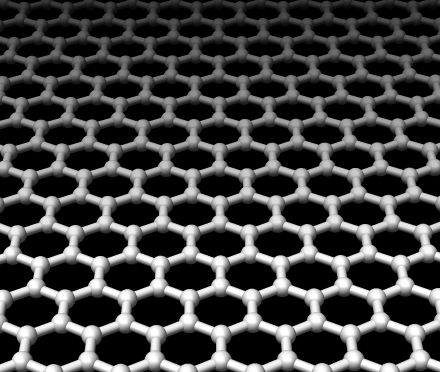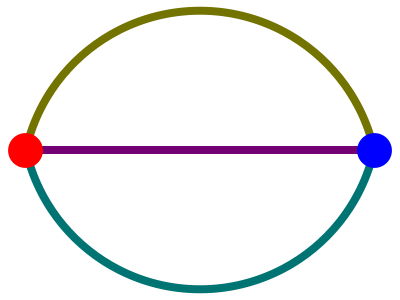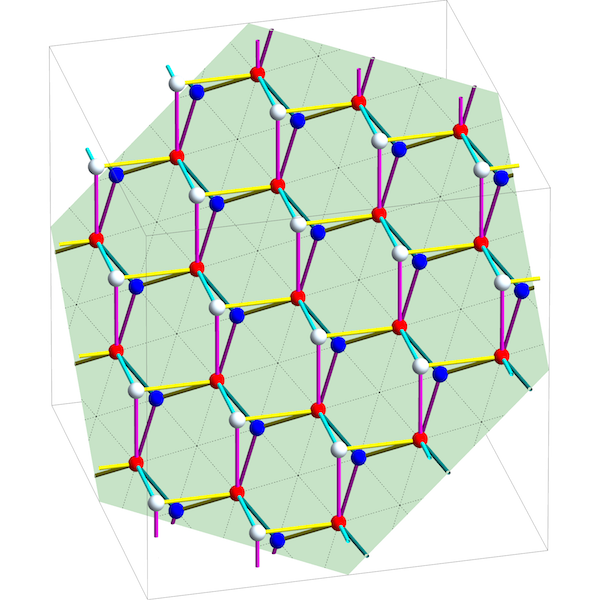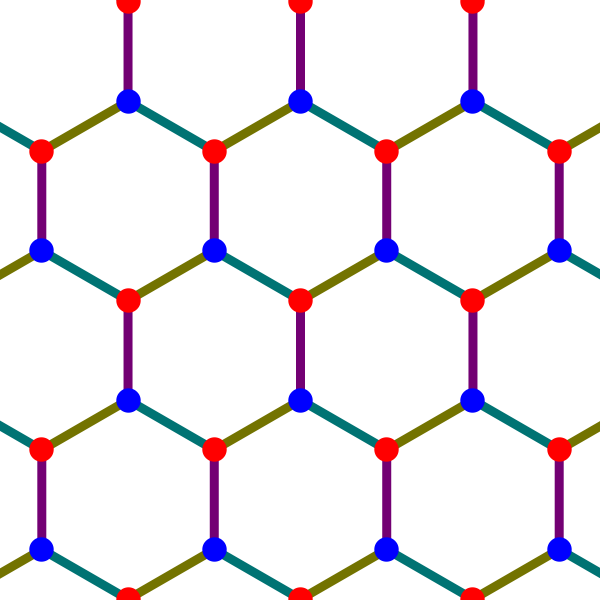July 23, 2016

Topological Crystals (Part 1)

Posted by John BaezOver on Azimuth I posted an article about crystals:

In the comments on that post, a bunch of us worked on some puzzles connected to ‘topological crystallography’—a subject that blends graph theory, topology and mathematical crystallography. You can learn more about that subject here:

I got so interested that I wrote this paper about it, with massive help from Greg Egan:

I’ll explain the basic ideas in a series of posts here.

First, a few personal words.

I feel a bit guilty putting so much work into this paper when I should be developing network theory to the point where it does our planet some good. I seem to need a certain amount of beautiful pure math to stay sane. But this project did at least teach me a lot about the topology of graphs.

For those not in the know, applying homology theory to graphs might sound fancy and interesting. For people who have studied a reasonable amount of topology, it probably sounds easy and boring. The first homology of a graph of genus $g$ is a free abelian group on $g$ generators: it’s a complete invariant of connected graphs up to homotopy equivalence. Case closed!

But there’s actually more to it, because studying graphs up to homotopy equivalence kills most of the fun. When we’re studying networks in real life we need a more refined outlook on graphs. So some aspects of this project might pay off, someday, in ways that have nothing to do with crystallography. But right now I’ll just talk about it as a fun self-contained set of puzzles.

I’ll start by quickly sketching how to construct topological crystals, and illustrate it with the example of graphene, a 2-dimensional form of carbon:I’ll precisely state our biggest result, which says when the construction gives a crystal where the atoms don’t bump into each other and the bonds between atoms don’t cross each other. Later I may come back and add detail, but for now you can find details in our paper.

Constructing topological crystals

The ‘maximal abelian cover’ of a graph plays a key role in Sunada’s work on topological crystallography. Just as the universal cover of a connected graph $X$ has the fundamental group $\pi_1(X)$ as its group of deck transformations, the maximal abelian cover, denoted $\overline{X}$, has the abelianization of $\pi_1(X)$ as its group of deck transformations. It thus covers every other connected cover of $X$ whose group of deck transformations is abelian. Since the abelianization of $\pi_1(X)$ is the first homology group $H_1(X,\mathbb{Z})$, there is a close connection between the maximal abelian cover and homology theory.

In our paper, Greg and I prove that for a large class of graphs, the maximal abelian cover can naturally be embedded in the vector space $H_1(X,\mathbb{R})$. We call this embedded copy of $\overline{X}$ a ‘topological crystal’. The symmetries of the original graph can be lifted to symmetries of its topological crystal, but the topological crystal also has an $n$-dimensional lattice of translational symmetries. In 2- and 3-dimensional examples, the topological crystal can serve as the blueprint for an actual crystal, with atoms at the vertices and bonds along the edges.

The general construction of topological crystals was developed by Kotani and Sunada, and later by Eon. Sunada uses ‘topological crystal’ for an even more general concept, but we only need a special case.

Here’s how it works. We start with a graph $X$. This has a space $C_0(X,\mathbb{R})$ of 0-chains, which are formal linear combinations of vertices, and a space $C_1(X,\mathbb{R})$ of 1-chains, which are formal linear combinations of edges. There is a boundary operator

$\partial \colon C_1(X,\mathbb{R}) \to C_0(X,\mathbb{R})$

This is the linear operator sending any edge to the difference of its two endpoints. The kernel of this operator is called the space of 1-cycles, $Z_1(X,\mathbb{R})$. There is an inner product on the space of 1-chains such that edges form an orthonormal basis. This determines an orthogonal projection

$\pi \colon C_1(X,\mathbb{R}) \to Z_1(X,\mathbb{R})$

For a graph, $Z_1(X,\mathbb{R})$ is isomorphic to the first homology group $H_1(X,\mathbb{R})$. So, to obtain the topological crystal of $X$, we need only embed its maximal abelian cover $\overline{X}$ in $Z_1(X,\mathbb{R})$. We do this by embedding $\overline{X}$ in $C_1(X,\mathbb{R})$ and then projecting it down via $\pi$.

To accomplish this, we need to fix a basepoint for $X$. Each path $\gamma$ in $X$ starting at this basepoint determines a 1-chain $c_\gamma$. These 1-chains correspond to the vertices of $\overline{X}$. The graph $\overline{X}$ has an edge from $c_\gamma$ to $c_{\gamma'}$ whenever the path $\gamma'$ is obtained by adding an extra edge to $\gamma$. This edge is a straight line segment from the point $c_\gamma$ to the point $c_{\gamma'}$.

The hard part is checking that the projection $\pi$ maps this copy of $\overline{X}$ into $Z_1(X,\mathbb{R})$ in a one-to-one manner. In Theorems 6 and 7 of our paper we prove that this happens precisely when the graph $X$ has no ‘bridges’: that is, edges whose removal would disconnect $X$.

Kotani and Sunada noted that this condition is necessary. That’s actually pretty easy to see. The challenge was to show that it’s sufficient! For this, our main technical tool is Lemma 5, which for any path $\gamma$ decomposes the 1-chain $c_\gamma$ into manageable pieces.

We call the resulting copy of $\overline{X}$ embedded in $Z_1(X,\mathbb{R})$ a topological crystal.

Let’s see how it works in an example!

Take $X$ to be this graph:Since $X$ has 3 edges, the space of 1-chains is 3-dimensional. Since $X$ has 2 holes, the space of 1-cycles is a 2-dimensional plane in this 3-dimensional space. If we take paths $\gamma$ in $X$ starting at the red vertex, form the 1-chains $c_\gamma$, and project them down to this plane, we obtain the following picture:Here the 1-chains $c_\gamma$ are the white and red dots. These are the vertices of $\overline{X}$, while the line segments between them are the edges of $\overline{X}$. Projecting these vertices and edges onto the plane of 1-cycles, we obtain the topological crystal for $X$. The blue dots come from projecting the white dots onto the plane of 1-cycles, while the red dots already lie on this plane. The resulting topological crystal provides the pattern for graphene:That’s all there is to the basic idea! But there’s a lot more to say about the mathematics it leads to, and a lot of fun examples to look at: diamonds, triamonds, hyperquartz and more.

Posted at July 23, 2016 8:18 AM UTC

TrackBack URL for this Entry:   https://golem.ph.utexas.edu/cgi-bin/MT-3.0/dxy-tb.fcgi/2890

Re: Topological Crystals (Part 1)

In email, Timothy Porter said this post reminded me of some stuff from a workshop in Bangor back in 2000, on Orbifolds and Crystallography. They invited Haefliger and also Carroll Johnson from Oak Ridge National Laborator, who has a website on this material:

The extended abstract for his Bangor talk is here.

My work with Egan is not directly related to orbifolds. From what I gather, the relevance of orbifolds is that you can take 3-dimensional space and mod out by the symmetry group of a crystal and get an orbifold. Of course you could just get a topological space, but orbifolds allow you to keep clear track of which points are fixed by more symmetries than the average point.

This works for any crystal. What Egan and I are doing is different. Following an idea of Sunada, we’re starting from any finite graph $X$ with no bridges—no edges whose removal disconnects the graph— and using this to build a crystal in $\mathbb{R}^n$, where $n$ is the genus of the graph. This crystal is itself a graph, with ‘atoms’ as vertices and ‘bonds’ as edges. And this graph is the maximal abelian cover of $X$.

Proving that we really get a copy of the maximal abelian cover embedded in $\mathbb{R}^n$ turns out to require some work. I’ll try to sketch how that goes.

And then there are various fun things you can do, like try to figure out various properties of this crystal depend on the original graph $X$.

Posted by: John Baez on July 24, 2016 7:23 AM | Permalink | Reply to this

Re: Topological Crystals (Part 1)

If as you say elsewhere:

In 1923, Hermann Weyl published a paper in Spanish which described electrical circuits in terms of the homology and cohomology of graphs (W). In this approach, Kirchhoff’s voltage and current laws simply say that voltage is a 1-coboundary and current is a 1-cocycle. Furthermore, the electrical resistances labelling edges of the graphs put an inner product on the space of 1-chains, allowing us to identify them with 1-cochains. Ohm’s law then says that voltage may then be identified with the current.

Is there any chance that your work points to some other feature of electricity?

Posted by: David Corfield on July 27, 2016 9:13 PM | Permalink | Reply to this

Re: Topological Crystals (Part 1)

There are big connections between topological crystallography and electrical circuit theory — if you read our original discussions you’ll see that Greg Egan and I used these connections a lot when developing our thoughts.

I later found this paper, which exploits this analogy intensively:

• J.-G. Eon, Euclidian embeddings of periodic nets: definition of a topologically induced complete set of geometric descriptors for crystal structures, Acta Crystallographica Section A 67 (2011), 68–86.

(Unfortunately mathematical crystallographers seem not to have embraced the arXiv, so there’s a lot of fascinating work that you can only access through Acta Crystallographica.)

When we got deeper into the subject, it became very important to think about integral chains and cochains. There are interesting refinements to the usual homology theory of graphs that kick in when we work over the integers rather than the real numbers. I’ll talk about this later.

Since nobody in electrical circuit theory demands that potentials or currents be integral, it’s hard to intuit what these refinements say about electricity! Instead, people use an analogy to ‘sandpiles’:

• Luis Garcia Puente, Dino Lorenzini, Criel Merino, David Perkinson and Carlos Enrique Valencia Oleta, Sandpile groups.
Posted by: John Baez on July 28, 2016 2:16 AM | Permalink | Reply to this

Re: Topological Crystals (Part 1)

Wow, that’s really very nice (and reminds me that I should look again at the excellent undergraduate text by Hirsch and Smale on differential equations) – but the linked page (John Baez, Circuit Theory I, at the ncatlab complex) appears to be blank.

Posted by: Todd Trimble on July 28, 2016 2:21 AM | Permalink | Reply to this

Re: Topological Crystals (Part 1)

Todd: I fixed David’s link. The correct link, to a very similar, URL, is this:

Weyl’s old work treats ‘closed’ circuits, without inputs and outputs, but this discusses more general ‘open’ circuits, where current can flow in or out at certain points. This brings relative cohomology into the game.

I’ve read that Weyl published his paper in Spanish because working on electrical circuits was considered too low-class for a pure mathematician such as him.

Posted by: John Baez on July 28, 2016 4:19 AM | Permalink | Reply to this

Re: Topological Crystals (Part 1)

It looks like whoever scanned that paper of Weyl’s was enjoying a tequila at the time, especially when they were doing page 5.

Posted by: Tom Leinster on August 3, 2016 10:48 PM | Permalink | Reply to this

Re: Topological Crystals (Part 1)

I remember one time you said that diamond was the closest you could get to the E8 lattice in three dimensions.

Posted by: Jeffery Winkler on July 28, 2016 12:38 AM | Permalink | Reply to this

Re: Topological Crystals (Part 1)

Yes, back in “week95” I wrote:

We start with the checkerboard lattice $\mathrm{D}_n$ in dimension $n$ — this is the lattice consisting of all integer-coordinate points whose coordinates add up to an even integer — and double its density by throwing in another copy of Dn shifted over by the vector $(\frac{1}{2}, \dots, \frac{1}{2})$. Conway and Sloan call the result $\mathrm{D}_n^+$. It’s only a lattice when $n$ is even, but when $n = 3$ it’s the pattern that carbon atoms form in a diamond! It’s only when we get up $n = 8$ that we can start with tightly packed spheres centered at the points of $\mathrm{D}_n$, and put in new spheres centered at the other points of $\mathrm{D}_n^+$ that are just as big, without having the spheres overlap. The result being $\mathrm{E}_8$, perhaps we could call $\mathrm{E}_8$ an “eight-dimensional diamond”, which at least conveys some of its crystalline beauty.

Later I learned a bit more and noticed that:

• $D_n^+$ is a lattice just when $n > 1$ is even. (It’s a lattice when the sum or difference of any two vectors in $D_n^+$ is again in $D_n^+$.)
• $D_n^+$ is an even lattice just when $n > 1$ is a multiple of 4. (Even means that the dot product of any vector in the lattice with itself is even.)
• $D_n^+$ is an even unimodular lattice just when $n > 1$ is a multiple of 8. (Unimodular means that the volume of each fundamental cell in the lattice is 1.)

Even unimodular lattices exist in $n$-dimensional space iff $n$ is a multiple of 8.

Posted by: John Baez on July 28, 2016 2:07 AM | Permalink | Reply to this

Re: Topological Crystals (Part 1)

I think what you are talking about is the embedding of the tropical curve (your graph) in the tropical Jacobian, which is $(\mathbb{R}/\mathbb{Z})^g$, where $g$ is $H^1$ of the graph, followed by taking the universal cover of the graph. See Mikhalkin-Zharkov or Baker-Faber.

Posted by: David Speyer on July 29, 2016 3:31 AM | Permalink | Reply to this

Re: Topological Crystals (Part 1)

Thanks! Let me see what’s going on. Theorem 1.8 of this paper

show that if $X$ is a bridgeless graph the vertices of $X$ are embedded in something they call the tropical Jacobian $Jac(X)$. They’re using a different definition of the Jacobian than you are: they say $Jac(X)$ is a finite abelian group whose order $\kappa(X)$ is the number of spanning trees in $X$. But I think this naturally embeds as a finite subgroup of your Jacobian. Right?

If so, this paper gives a different proof of what’s called Theorem 2 in my paper — and one that seems to contain a lot of the same ideas as the argument you gave for Lemma 6, the key step to proving that theorem.

I wound up not using your argument, because a different argument seemed better for proving Theorem 7. This stronger result says that not only the vertices but the whole graph $G$ is embedded in your tropical Jacobian $(\mathbb{R}/\mathbb{Z})^g$. That is, no edges cross each other.

This paper discusses the edges:

Theorem 4.1 says that each edge, individually, is embedded. In fact, it’s embedded isometrically if we put some natural metric on the graph $G$ and the torus $(\mathbb{R}/\mathbb{Z})^g$. However, it still doesn’t prove that different edges don’t cross each other!

I pointed this out to Matt and he agreed. He said everyone who works on tropical curves and chip firing knows that the edges don’t cross. He said it’s a “simple consequence of Dhar’s burning algorithm plus the fact that a $q$-reduced divisor D on a metric graph is equivalent to an effective divisor if and only if $D(q) \geq 0$”. He said there must be a reference for this result. However, I still haven’t seen one.

Do you know one?

Needless to say, I like the proof Greg Egan and I came up with: not only because we sweated a lot to get it, but because it relies on a very pretty fact about 1-chains on graphs, Lemma 5.

Posted by: John Baez on August 6, 2016 8:40 AM | Permalink | Reply to this

Post a New Comment